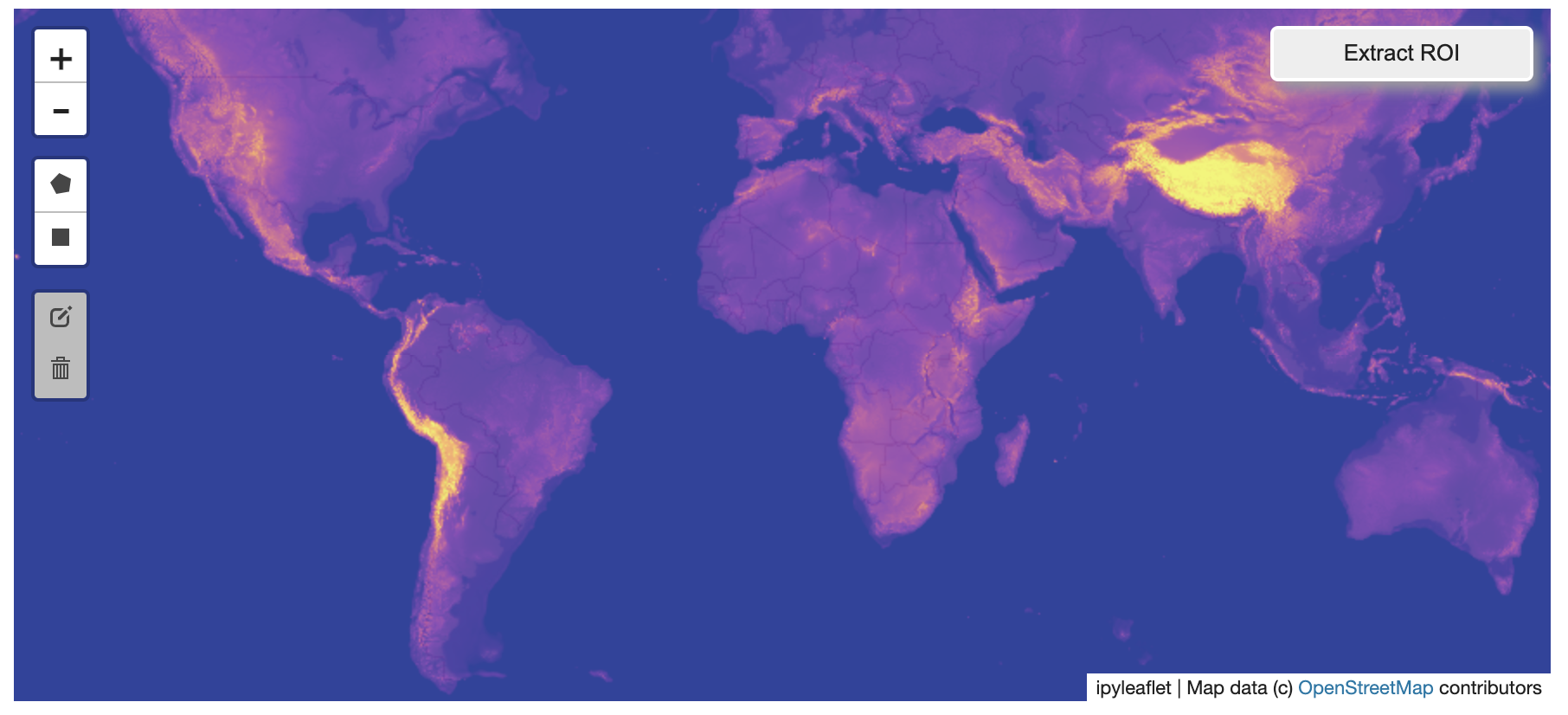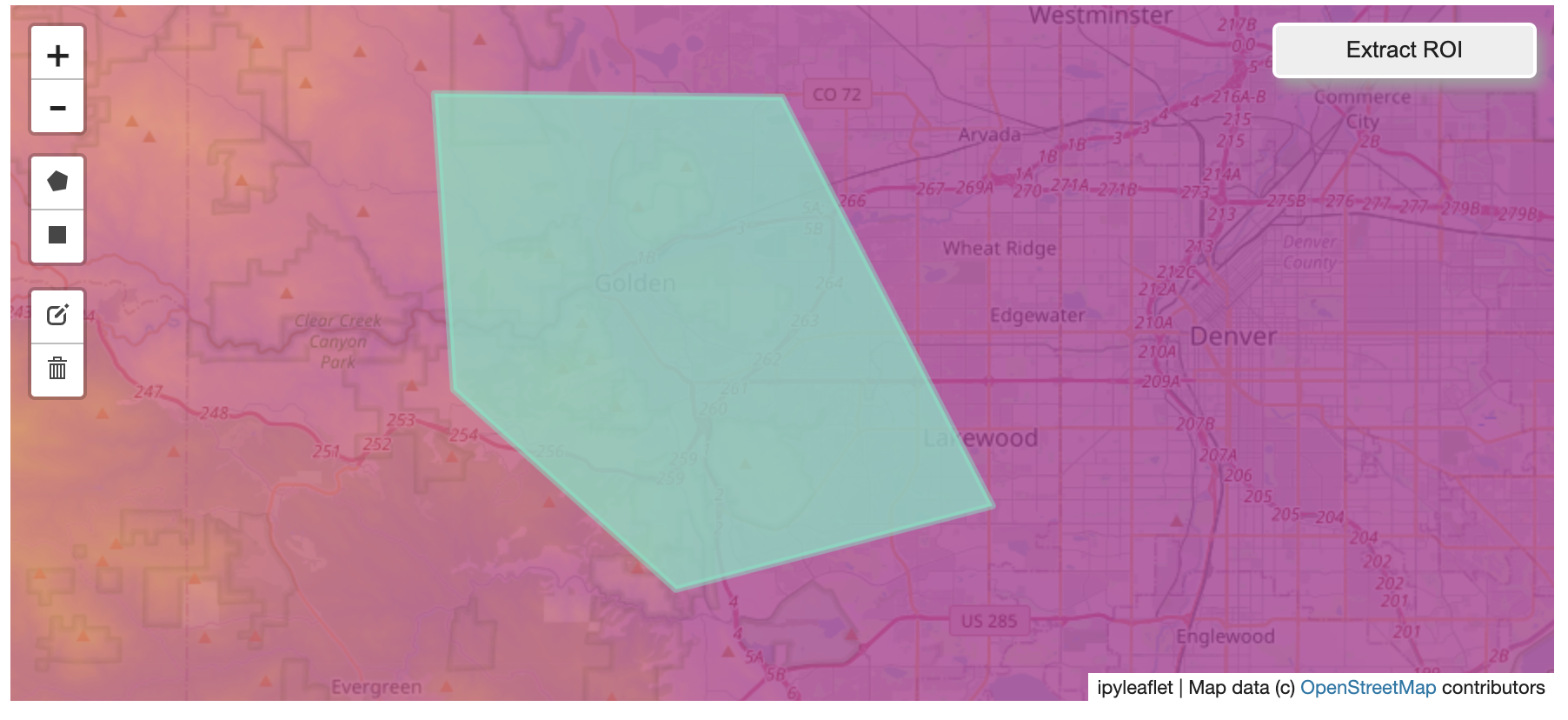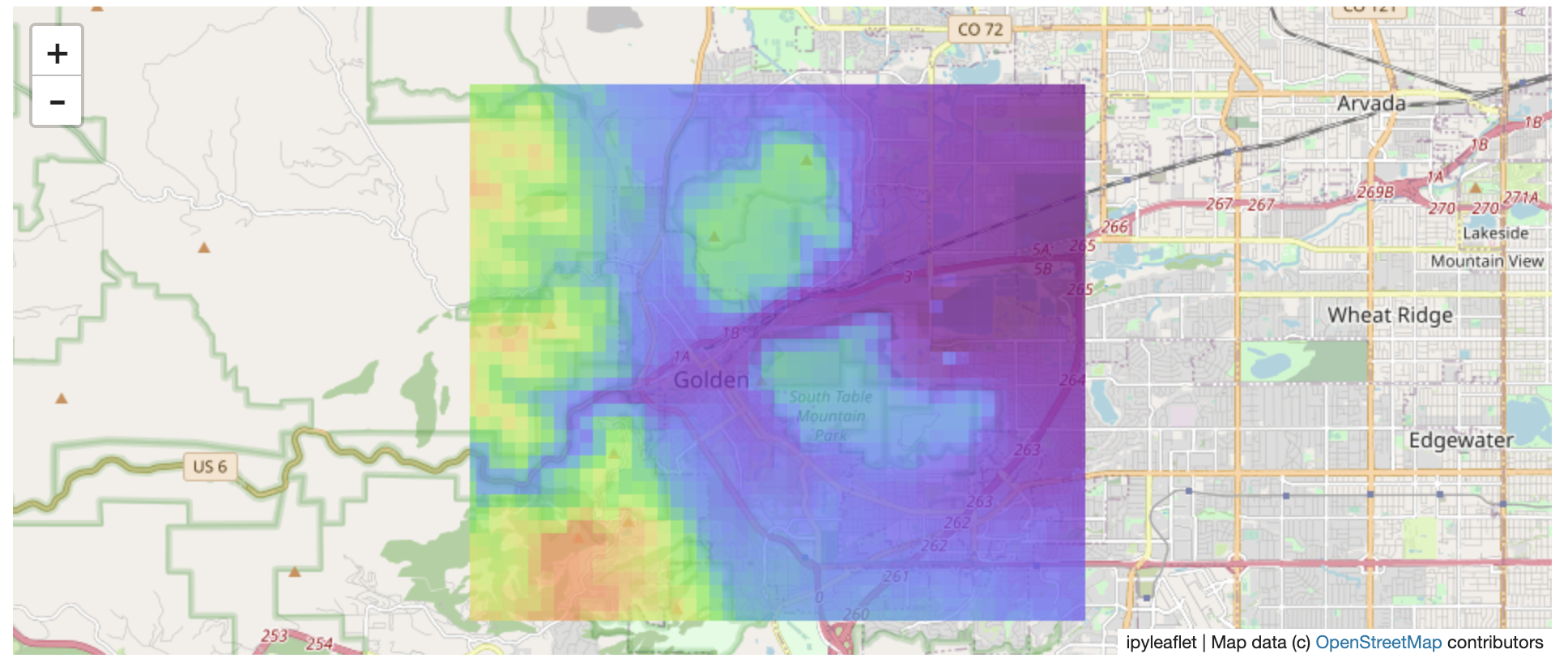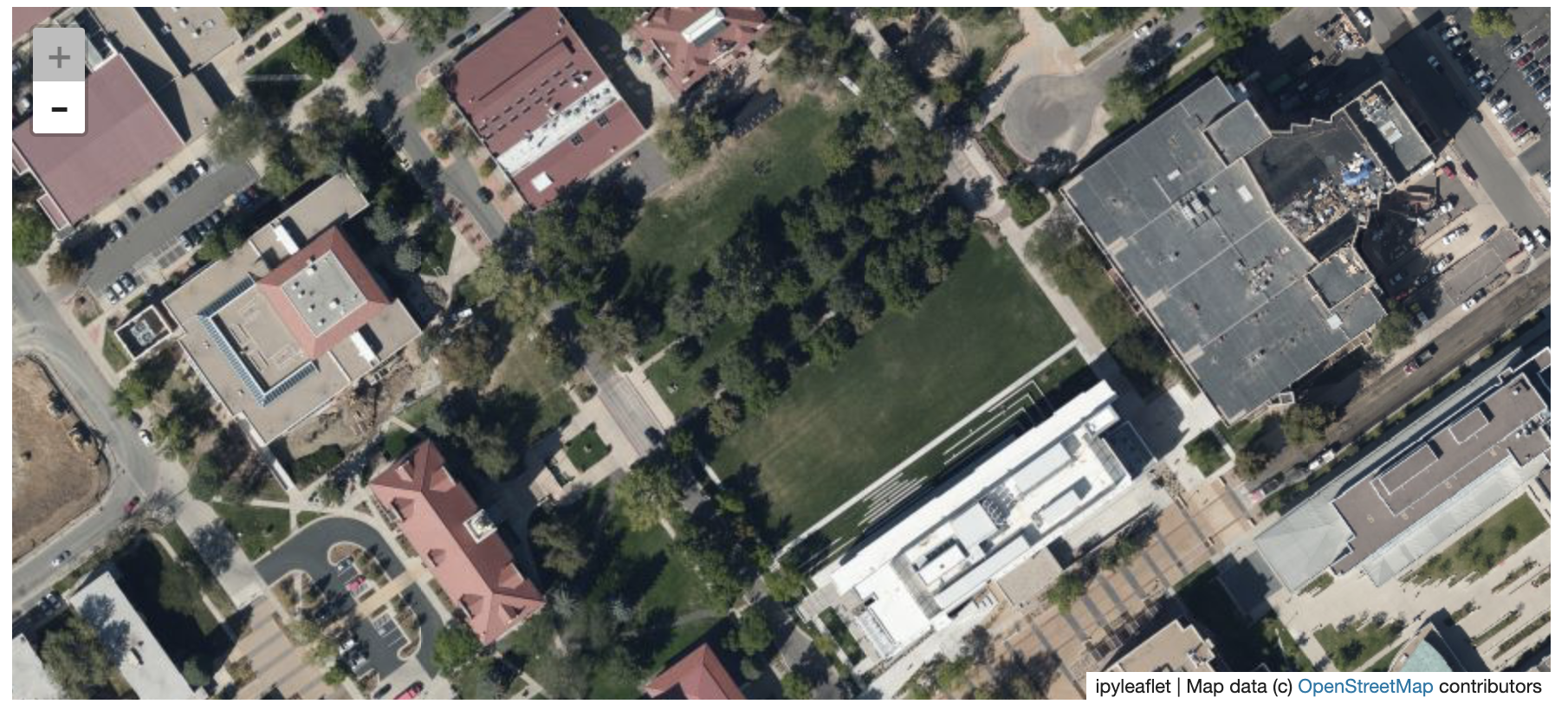# 🗺️ Example Datasets#

A few example datasets are included with localtileserver. A particularly useful one has global elevation data which you can use to create high resolution Digital Elevation Models (DEMs) of a local region.

```from localtileserver import get_leaflet_tile_layer, get_leaflet_roi_controls, examples
from ipyleaflet import Map

# Load example tile layer from publicly available DEM source
client = examples.get_elevation()

# Create ipyleaflet tile layer from that server
t = get_leaflet_tile_layer(client,
band=1, vmin=-500, vmax=5000,
palette='plasma',
opacity=0.75)

# Create ipyleaflet controls to extract an ROI
draw_control, roi_control = get_leaflet_roi_controls(client)

m = Map(zoom=2)
m
```Then you can follow the same routine as described above to extract an ROI.

I zoomed in over Golden, Colorado and drew a polygon of the extent of the DEM I would like to create:And perform the extraction:

```roi_path = '...'  # Look in your working directory

r = get_leaflet_tile_layer(roi_path, band=1,
palette='plasma', opacity=0.75)

m2 = Map(
center=(39.763427033262175, -105.20614908076823),
zoom=12,
)
m2
```Here is another example with the Virtual Earth satellite imagery

```from localtileserver import get_leaflet_tile_layer, examples
from ipyleaflet import Map

# Load example tile layer from publicly available imagery
client = examples.get_virtual_earth()

# Create ipyleaflet tile layer from that server
t = get_leaflet_tile_layer(client, opacity=1)

m = Map(center=(39.751343612695145, -105.22181306125279), zoom=18)